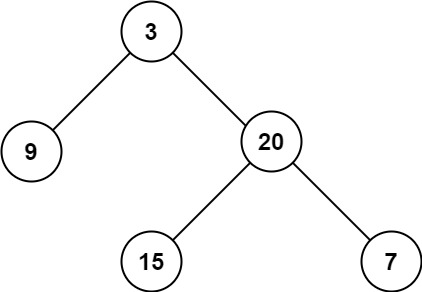# GeetCode Hub

Given the `root` of a binary tree, return its maximum depth.

A binary tree's maximum depth is the number of nodes along the longest path from the root node down to the farthest leaf node.

Example 1:```Input: root = [3,9,20,null,null,15,7]
Output: 3
```

Example 2:

```Input: root = [1,null,2]
Output: 2
```

Example 3:

```Input: root = []
Output: 0
```

Example 4:

```Input: root = 
Output: 1
```

Constraints:

• The number of nodes in the tree is in the range `[0, 104]`.
• `-100 <= Node.val <= 100`

/** * Definition for a binary tree node. * public class TreeNode { * int val; * TreeNode left; * TreeNode right; * TreeNode() {} * TreeNode(int val) { this.val = val; } * TreeNode(int val, TreeNode left, TreeNode right) { * this.val = val; * this.left = left; * this.right = right; * } * } */ class Solution { public int maxDepth(TreeNode root) { } }